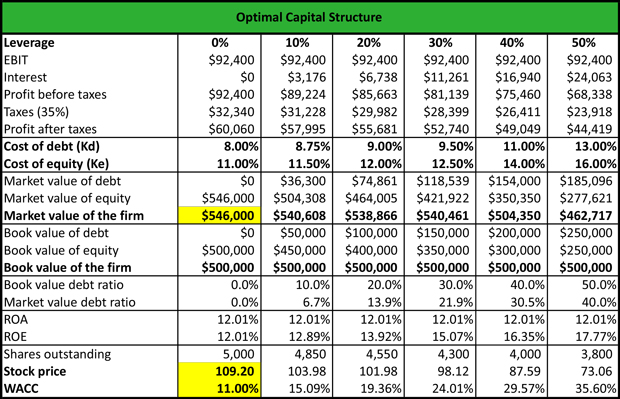# What is Optimal Capital Structure?

Definition: Optimal capital structure is a financial measurement that firms use to determine the best mix of debt and equity financing to use for operations and expansions. This structure seeks to lower the cost of capital so that a firm is less dependent on creditors and more able to finance its core operations through equity.

## What Does Optimal Capital Structure Mean?

What is the definition of optimal capital structure? In general, the optimal capital structure is a mix of debt and equity that seeks to lower the cost of capital and maximize the value of the firm. To calculate the optimal capital structure of a firm, analysts calculate the weighted average cost of capital (WACC) to determine the level of risk that makes the expected return on capital greater than the cost of capital.

By calculating the cost of debt and the cost of equity, analysts multiply the cost of debt by the weighted average cost of debt and the cost of equity by the weighted average cost of equity and add up the results from each security involved in the total capital of the company.

Let’s look at an example.

## Example

Jenny works as a financial analyst at Morgan Stanley. She is asked to create an optimal capital structure spreadsheet that contains different leverage, interest expenses, tax expenses with a tax rate 35%, the cost of debt, and the cost of equity. Based on the inputs, Jenny calculates the market value of the firm and the book value of the firm, as follows:The different leverage does not have an impact on the company’s EBIT. However, as the cost of debt increases from 8.00% to 13.00% and the market value of debt increases from \$36,300 to \$185,096, the interest expenses increase and the profits after tax decline.

The cost of debt and the cost of equity indicate the required return on debt and on equity so that investors buy the company’s stock. As the leverage increases from 0% to 50%, the cost of debt and the cost of equity increase, respectively, suggesting that investors require a higher return to undertake a higher risk.

Jamie concludes that a WACC of 11.00% maximizes the firm’s value (\$546,000), and the stock price (109.20) at the lowest cost of capital.

## Summary Definition:

Define Optimal Capital Structure: OCS determines the debt-to-equity ratio that maximizes a firm’s value by balancing debt and equity financing.